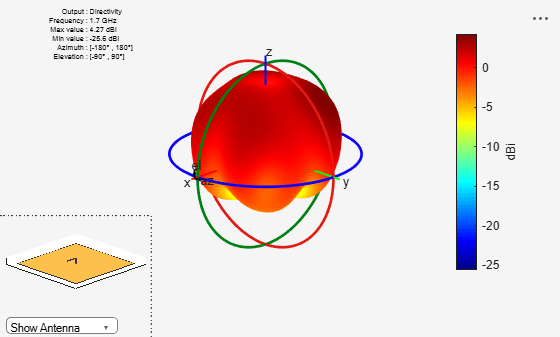Documentation

# invertedL

Create inverted-L antenna over rectangular ground plane

## Description

The `invertedL` object is an inverted-L antenna mounted over a rectangular ground plane.

The width of the metal strip is related to the diameter of an equivalent cylinder by the equation

`$w=2d=4r$`

where:

• d = diameter of equivalent cylinder

• a = radius of equivalent cylinder

For a given cylinder radius, use the `cylinder2strip` utility function to calculate the equivalent width. The default inverted-L antenna is center-fed. The feed point coincides with the origin. The origin is located on the X-Y plane.## Creation

### Syntax

``l = invertedL``
``l = invertedL(Name,Value)``

### Description

``` `l = invertedL` creates an inverted-L antenna mounted over a rectangular ground plane. By default, the dimensions are chosen for an operating frequency of 1.7 GHz.```

example

``` `l = invertedL(Name,Value)` creates an inverted-L antenna, with additional properties specified by one or more name-value pair arguments. `Name` is the property name and `Value` is the corresponding value. You can specify several name-value pair arguments in any order as `Name1`, `Value1`, `...`, `NameN`, `ValueN`. Properties not specified retain their default values.```

## Properties

expand all

Height of inverted element along z-axis, specified a scalar in meters.

Example: `'Height',3`

Data Types: `double`

Strip width, specified as a scalar in meters.

### Note

Strip width should be less than `'Height'`/4 and greater than `'Height'`/1001. 

Example: `'Width',0.05`

Data Types: `double`

Stub length along x-axis, specified as a scalar in meters.

Example: `'Length',0.01`

Ground plane length along x-axis, specified a scalar in meters. Setting `'GroundPlaneLength'` to `Inf`, uses the infinite ground plane technique for antenna analysis.

Example: `'GroundPlaneLength',4`

Data Types: `double`

Ground plane width along y-axis, specified as a scalar in meters. Setting `'GroundPlaneWidth'` to `Inf`, uses the infinite ground plane technique for antenna analysis.

Example: `'GroundPlaneWidth',2.5`

Data Types: `double`

Signed distance from center along length and width of ground plane, specified as a two-element vector.

Example: `'FeedOffset',[2 1]`

Data Types: `double`

Lumped elements added to the antenna feed, specified as a lumped element object handle. For more information, see `lumpedElement`.

Example: `'Load',lumpedelement`. `lumpedelement` is the object handle for the load created using `lumpedElement`.

Example: ```l.Load = lumpedElement('Impedance',75)```

Tilt angle of the antenna, specified as a scalar or vector with each element unit in degrees. For more information, see Rotate Antennas and Arrays.

Example: `'Tilt',90`

Example: `'Tilt',[90 90]`,`'TiltAxis',[0 1 0;0 1 1]` tilts the antenna at 90 degree about two axes, defined by vectors.

Data Types: `double`

Tilt axis of the antenna, specified as:

• Three-element vectors of Cartesian coordinates in meters. In this case, each vector starts at the origin and lies along the specified points on the X-, Y-, and Z-axes.

• Two points in space, each specified as three-element vectors of Cartesian coordinates. In this case, the antenna rotates around the line joining the two points in space.

• A string input describing simple rotations around one of the principal axes, 'X', 'Y', or 'Z'.

Example: `'TiltAxis',[0 1 0]`

Example: `'TiltAxis',[0 0 0;0 1 0]`

Example: `ant.TiltAxis = 'Z'`

## Object Functions

 `show` Display antenna or array structure; Display shape as filled patch `info` Display information about antenna or array `axialRatio` Axial ratio of antenna `beamwidth` Beamwidth of antenna `charge` Charge distribution on metal or dielectric antenna or array surface `current` Current distribution on metal or dielectric antenna or array surface `design` Design prototype antenna or arrays for resonance at specified frequency `EHfields` Electric and magnetic fields of antennas; Embedded electric and magnetic fields of antenna element in arrays `impedance` Input impedance of antenna; scan impedance of array `mesh` Mesh properties of metal or dielectric antenna or array structure `meshconfig` Change mesh mode of antenna structure `pattern` Radiation pattern and phase of antenna or array; Embedded pattern of antenna element in array `patternAzimuth` Azimuth pattern of antenna or array `patternElevation` Elevation pattern of antenna or array `returnLoss` Return loss of antenna; scan return loss of array `sparameters` S-parameter object `vswr` Voltage standing wave ratio of antenna

## Examples

collapse all

Create and view an inverted-L antenna that has 30mm length over a ground plane of dimensions 200mmx200mm.

```il = invertedL('Length',30e-3, 'GroundPlaneLength',200e-3,... 'GroundPlaneWidth',200e-3); show(il)```Plot the radiation pattern of an inverted-L at a frequency of 1.7GHz.

```iL = invertedL('Length',30e-3, 'GroundPlaneLength',200e-3,... 'GroundPlaneWidth',200e-3); pattern(iL,1.7e9)```Balanis, C.A. Antenna Theory. Analysis and Design, 3rd Ed. New York: Wiley, 2005.

 Volakis, John. Antenna Engineering Handbook, 4th Ed. New York: Mcgraw-Hill, 2007.